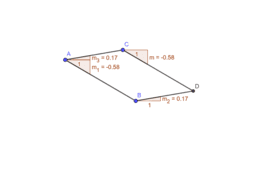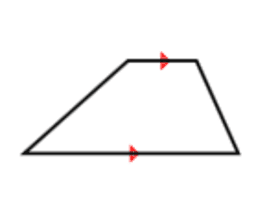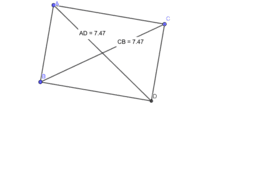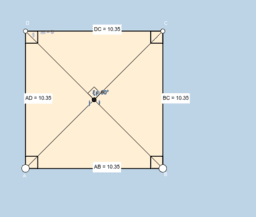# Parallelogram, Rectangle, and Square

Topic:
Geometry
With this activity students will be able to articulate the four definitions of a parallelogram: opposite sides are parallel, opposite sides are congruent, opposite angles are congruent, diagonals bisect each other and use the special characteristics of parallelograms when given a rectangle, rhombus, or square.
•### Four Definitions of a Parallelogram

•### Identify Parallelograms

•### Rectangle is a Special Kind of a Parallelogram

•### Rectangle Undersatanding

•